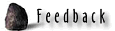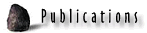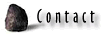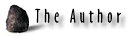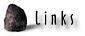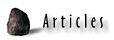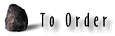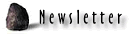THE FEW SET ASIDE TO BLESS THE MANY.

Under the age-old system, the brightest and most adept children were taken in as initiates into the advanced Schools of Learning, where Masters of the Craft would teach them skills much needed by regional society.
In Britain alone, there were 40 Druidic Schools of Learning and 60 universities, when the Roman's first made their unwelcome incursion into British affairs and, within the centuries to follow, highly developed scholarship was supplanted by Romanised brutish ignorance.

The British schools had been a part of a continuous system that had persisted for millennia, with a legacy extending into the Near East and beyond, into the forgotten mists of time.
The horseshoe component of the Octagon was, long ago, such a school, where alert young minds, under the guidance of mature tutors, learned the precious arts.

On this spot, astronomer/ navigator/ mathematicians were told how large the Earth was and taught how to grid reference it for journeys to the furthest reaches of the globe. They were taught how to predict weather patterns, by knowing how the moon was positioned relative to the Earth and from whence the winds could be predicted to come...or how to predict dangerous seasons in scattered climes anywhere upon the face of the globe.
They learned about the zenith stars that sat directly above countries at midnight, on particular nights of the year or how to read latitude precisely from shadows caste by the sun at midday...and so, so much more.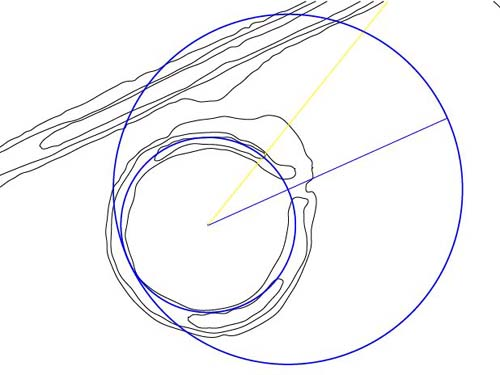Figure 77: How navigation was taught at the horseshoe "School of Learning". The same method was employed as that used within the greater Octagon complex, wherein the fulcrum or hub point for observation was in the gateway. The two circles depicted are 1/10th the size of those used from all 8 gateways of the main complex.

• The circle determining where the observer would stand was based upon 2 X 1/2 PHI expansions of the number related to Khafre Pyramid's height (at 1/10th scale)...47.25 feet radius ...expanded in increments of .80901695 (1/2 PHI) to 72.19158405 feet radius or 144.3831681 feet diameter.
• This value was then multiplied by PI (3.1416) to deliver up a circumference length of 453.6 feet (the exact sexagesimal height assignment of the Great Pyramid to its flat top altar floor).
• The circle of 453.6 feet circumference had amazing, versatile attributes, which allowed it to be pressed into service for many varying calibrations using several different measurement increments. It could be used for solar, lunar, stellar, Sabbatical calendar or navigational endeavours simultaneously.
• The 453.6 feet was read, also, as 453.049492 feet (pure PHI rendition and exact true intended height of the Great Pyramid). All calculations involving the PHI inch, PHI foot or Megalithic Yard used the pure PHI assignment.
• The circle was also read as 453.75 feet when making calculations under the mantel of the "11" series, geodetic, Earth navigational system, using leagues, miles, furlongs and related increments.

Let's begin with stellar angle determinations, using the 453.6 feet assignment: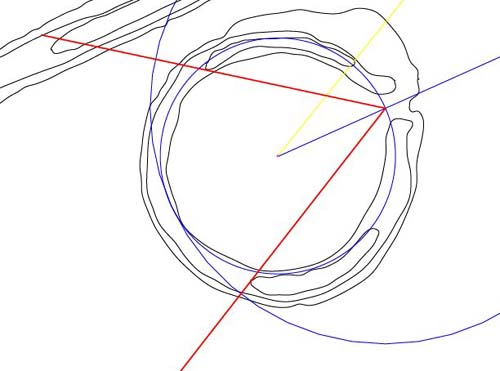Figure 78: From the entryway station, the two red vectors are sent out, which brush the nose sections of the crest mounds. The range of azimuth covered by this sweep is 64-degrees. If one utilised the larger of the two circles for the calibration ring, then each degree of arc would equate to 2.52 feet or 30.24 inches. Note: the Great Pyramid was 3024 feet in perimeter.

Alternatively, one could use the smaller circle (by moving the observer's station to the centre) @ 15.12 inches per degree of arc. Remember, the distance between the centre of the Octagon's circle embankment and the centre of the horseshoe is 1512 feet.

• The two circles (based upon 453.6 feet & 907.2) could be calibrated in expressions of the reed, Celtic Royal Cubit, Celtic common cubit or rounded megalithic yard. The so-called, Egyptian Royal Cubit (20.61818182 inches or common cubit (17.67272727 inches) could also be used.
• Under its reed assignment the circumference of the larger circle was 86.4 reeds. This value is in homage to the sun and the Sun God RA, inasmuch as the sun is 864,000 miles in diameter. One degree of arc on this circle was .24 of a reed.
• Using the smaller circle, the reed value is reduced to 43.2. This provides a formula for determining the size of the Earth, which was to multiply the perimeter value of the Great Pyramid (under any one of 3 assignments ...3024 feet, 3025 feet or 1120 MY) X 43200 to get the size of the Earth under that assignment. In each case, the perimeter value of the Great Pyramid represented 1/2 a minute of arc for the world.
• If the larger circle were read in Celtic Royal Cubits of 21 inches each, then the sum total for the sweep would be 518.4. This codes the face angle of the Great Pyramid @ 51.84 degrees, which is the same azimuth angle that the Octagon lies on from its altar, through its Avenue to its north-eastern gateway. The number 51.84 or enlargements of it were of extreme importance to navigation. For example: the linear distance of 1 league (16500 feet) converts to a circle of 51840 feet (using the 3.141818182 rendition of PI). A 500th part of precession is 51.84 years.
• The Great Pyramid @ 72 reeds per side (756 feet) was 5184 square reeds in base area. Its base and sides Egyptian acreage total was 51.845.
• Using the smaller circle... its Celtic Royal Cubit circumference is 259.2. The precession of the equinoxes endured for 25920 years. The sun moves through a house of the zodiac, as read at the time of the vernal equinox, 1-degree every 72 years. A degree of arc, under this circle calibration is .72 of a Celtic Royal Cubit.
• If the larger circle were read as Celtic common cubits of 18 inches, then the total would be 604.8. One minute of arc, under the sexagesimal geodetic assignment was 6048 feet. If the smaller circle was read under this incremental value then it would equate to 302.4 Celtic common cubits...the Great Pyramid was 3024 feet in perimeter.
• The small circle's rounded megalithic yard value, 168 is indicative of the height of the Great Pyramid in rounded MY's. The number was anciently quite popular for 3,4,5 triangulation, using an opposite of 168.
• The 453.6 feet value is 264 Egyptian Royal Cubits of 20.61818182 inches or 308 Egyptian common cubits of 17.67272727 inches. The larger circle equates to 528 Egyptian Royals' and 616 commons'.

The forgoing would teach initiates how to work with circles that broke down by sexagesimal and septimal increments and how to make azimuth angle determinations within 360-degrees or 720 divisions.

NAVIGATION

The 453.6 feet circle would also be represented as both 453.049492 (280 PHI feet...1 foot X 1.6180339) and 453.75 feet (82.5 fathoms). It was of extreme importance that initiates learn about the 3 main geodetic navigational systems and that the horseshoe's navigational circle, regardless of the marginally different assignments, was 1/288000th of the ring of the Earth.
Tutorials would have included how the Earth can be grid referenced in degree increments, based upon the number 6 or leagues, based upon an "11" series of numbers.

THE MOON

The number 453.6 works very well with the lunar nutation cycle number, 6804. If the 453.6 feet are reduced to inches, then the total is 5443.2. This value divided by 68.04 = 80. Alternatively, 6804 is 453.6 X 15.

THE SABBATICAL CALENDAR & SOLAR YEAR COUNTS

At Stonehenge, the Sabbatical calendar system, used for staying abreast of the solar year, was worked out by counting through 56 post-positions on the Aubrey Circle. This system also worked perfectly for the lunar nutation cycle, through counts of either 56 or 54 positions.
The 453.6 feet circle lends itself admirably to counts of either 54 or 56. It is 8.1 feet (a strong lunar code) X 56 or 8.4 feet (a strong solar code) X 54. The base perimeter of the Great Pyramid was 56 X 54 feet.

THE ANCHOR ALIGNMENT TO THE ESE EMBANKMENT & THE ENTRYWAY BENCHMARK

The alignment up the crest the ESE embankment from the horseshoe hub is 38.88-degrees and, as discussed, this is indicative of a mathematical progression founded upon 6.48 & 12.96 (precession). Alternatively, the benchmark alignment, possibly based upon the rise position of the moon at minor northern standstill, is set to an azimuth of 66-degrees, designated by the centre of the doorway. The 66-degree value codes numbers related to the "11" series used for navigation.

By subtle redefinition, the 38.88-degrees could symbolically represent 38.57142857-degrees, which is simply a "7th" based expression within 360-degrees. Alternatively, the 66-degree entryway position could be substituted for 64.28571425-degrees.

The difficult numbers generated are indicative of dividing a 360-degree circle into 56 positions (a much practiced ancient modus operandi). The 453.6 feet circuit equates to 7 segments of 64.8 feet (precession).
The tutorial would have included a component that taught initiates how to create a calibrated circle based upon 630 instead of 360, such that difficult 7th increments were converted to simple expressions of 90.

By using the 1.75 to 1 ratio difference between 630 & 360, the difficult number 38.57142857 in a 360 circuit would convert to 67.5 in a 630 circuit. Likewise, 64.28571425 would convert to 112.5. By such means, circles could be read easily in sevenths, using more simplified and workable numbers.

3,4,5 TRIANGULATIONFigure 79: One of the most important geometric principles to be taught to initiates was 3,4,5 triangulation. The way that the horseshoe is configured lends itself admirable to this task and, by all appearances, 3,4,5 triangles with adjacent side values ranging from 68.04 feet to, conceivably, 88 feet were being demonstrated.
In the above picture the 3,4,5 has an adjacent of 75.6 feet, an opposite of 100.8 feet and a hypotenuse of 126 feet.

• The 75.6 feet adjacent runs from the hub of the horseshoe to the nose section of the crescent hump situated atop the southern section of embankment. Although some slumping of the nose appears to have occurred in the millenniums of abandonment, there remains something very close to a 90-degree offset between this nose and that of the northern crescent's eastern nose section.
• The distance of 75.6 feet is in deference to the length of the Great Pyramid, which was 756 feet. Note how this adjacent extends to the centre line of the crescent.
• The opposite is 100.8 feet and lies on the horseshoe's anchor line at 38.88-degrees azimuth. It brushes the nose en route to its point of termination upon the raised terrace to the exterior of the horseshoe. The distance of 100.8 feet is exactly 1-second of arc for the World under the sexagesimal geodetic navigational system. This same measurement was built into the Sarsen Circle at Stonehenge, where the inner faces of the lintels (in one cross measurement) equated to 100.8 feet.
• The outer terrace appears to have been a place where "crossing strings" running from the hub & adjacent to the opposite, formed the hypotenuse. Since a variety of specialised 3,4,5's were being created and their merits elucidated upon, it was important to have an expanse of terrain upon which an alignment pole, indicating the point of intersection, could be set.
• Note how the terrace extends outward on this azimuth, as if designed to provide space for a variety of 3,4,5's.
• Note how the 100.8 feet radius circle swirls to the main SSE embankment of the Octagon, where a peninsula feature of additional embankment extends toward the brushing circle.
• The hypotenuse of this 3.4.5 is 126 feet (12 reeds or 1/5th of a Greek stadium measurement).

ALTAR TO INNER MOUND CODES

At the rear section of the altar is the primary station position, which equated to 2025 feet (750 rounded MY or 24300 inches) from the hub of the horseshoe.
This position appears to carry a dual code, as it would be useful to symbolically represent it as 2133.3333 feet (25600 inches) from the hub position of the Octagon, whereas it is, literally, 2134.8 feet.
The 2025 feet represent a value within a lunar and rounded PHI progression...especially in its inch count of 24300.
The lunar nutation period was 28 X 243 days.

The 2133.3333 feet (symbolic) is simply 1/3rd of 6400...a much used number in ancient astronomy...8 X 8 and the main number (64) adopted by the Knights Templar.
From this position, many stations across the expanse of the Octagon had significance in coded lengths and angles. In the interests of brevity, only some of these will be identified at this time and researchers are encouraged to extract the rest at their leisure.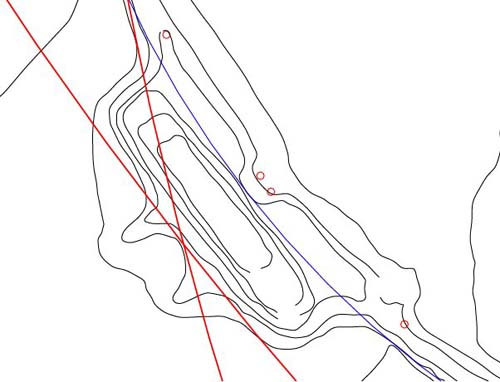Figure 80: The crossing position of a circle of 2025 feet, centred upon the hub of the horseshoe, intersecting with one of 2133.3333 feet (symbolic) but 2134.8 actual from the hub of the Octagon. From this position crown points on the small inner mounds of the Octagon had significance in both distance and angle.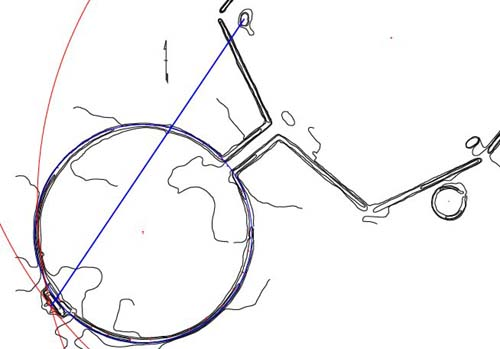Figure 81: The blue vector runs from the rear of the altar to the small mound adjacent to the western gateway. The length of the run to the crown of the small embankment is 1701 feet. The azimuth angle of the vector is 340.2-degrees. Note how the vector crosses the customised peninsula humps as it traverses the circle embankment edge. That interim position between points is also carefully coded.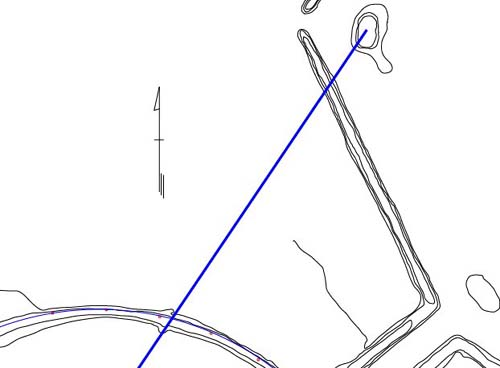Figure 82: The blue vector traversing the rim of the circle embankment en route to its point of termination atop the small inner mound. Note how the vector relates to the peninsula humps, deliberately built into the embankment rim to accentuate this important alignment, which codes the lunar nutation cycle.

• The distance between the rear of the altar to the crown of the small mound is 1701 feet & this codes 1701 days, which is 1/4th of the 6804 day lunar nutation cycle.
• The angle between the 2 points is 340.2-degrees and this codes 3024 days, which is 1/2 of the lunar nutation cycle.
• The distance to a prominent, second nose position upon the inner peninsula is 1036.8 feet, which is 1/5th of 5184...the site lies on an azimuth of 51.84-degrees and the linear distance of 1 ancient league (16500 feet) converts to a circumference of 51840 feet (divisible by 360-degrees and a principle used in ancient navigation). A circle sent out from the altar and sized to this radius swirls across the site and runs through a notch on the inner bank at the opposite side of the circle embankment. To understand one expression of the 1036.8 code, it must be realised that the ancient formula for the true equatorial circumference of the Earth was 12 X 12 X 12 X 12 X 1.2 = 24883.2 miles. Therefore, at the rate of one rotation each 24-hours, the speed of the Earth's rotation is 1036.8 miles per hour.
• Codes for 1050 feet (100 reeds) and 1056 feet (1/5th of a mile) seem also to be present between the altar station and the embankment peninsula station. These codes were most certainly encrypted into the embankment circle across its full diameter, with the crest to crest width measured by Illinois surveyor, James A. Marshall, as 1056 feet.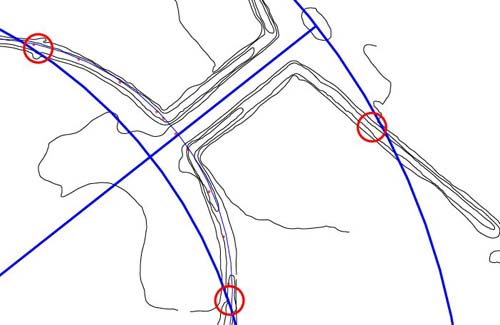Figure 83: The coded distance to the small mound at the end of the Avenue is 1485 feet (550 rounded megalithic yards), which also happens to be an exterior defining limit of the octagonal component of the site. The azimuth angle of this vector remains 51.84-degrees. The small red circles show where features appear, strongly, to have been built into the embankments for encrypting circle and linear codes. The lesser circle, marked by opposing, inbuilt features to each side of the circle embankment, codes 1036.8 feet in its radius, which is 1/5th of 5184 feet.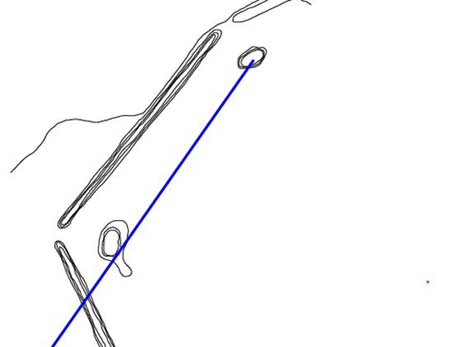Figure 84: The vector from the altar station runs out for 2227.5 feet, which is 825 rounded megalithic yards of 32.4 inches each. This code is in deference to 1650 (2 X 825), which was at the basis of the league measurement. The apparent angle being coded is 35-degrees...or a 7 based mathematical progression.

Other degree angle coding on marked positions of the mound would have included 34.56-degrees to the left side of the mound (Stonehenge's outer rim on the Sarsen circle was 345.6 feet) and 34.5375-degrees to the right (the lunar year is 345.375 days).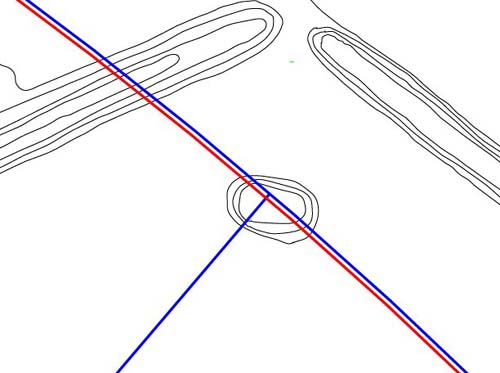Figure 85: This position effectively demonstrates both rounded PHI and pure PHI values. The blue line is set 2700 feet from the altar station or 1666.6666 rounded PHI feet of 1.62 feet each. The blue circle is slightly forward of the mound's centre. The red circle is 1666.6666 pure PHI feet of 1.6180339 feet each and better represents the centre position. The angle to this small mound appears to be coded to 40.5-degrees, which is a value within a strong lunar mathematical progression. Also found within the progression are rounded PHI and precessional numbers.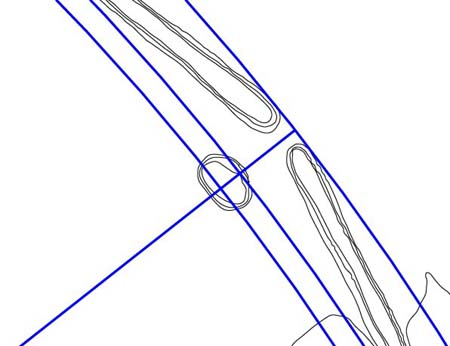Figure 86: Two very strongly coded positions falling onto the small mound adjacent to the northeast gateway, with another dynamic code brushing the exterior of the site. The most inward circle edge is 2772 feet from the altar station and this value is, simultaneously, 264 reeds (mile) or 504 fathoms (Great Pyramid).

The other circle crossing the forward position of the mound is 2800 feet from the altar station. This value simply codes the multipurpose number 28, used in solar & lunar determinations and central to the Sabbatical calendar.

A very important code is found in the circle edge brushing the outer line of the embankments. This limit is 2880 feet from the altar station and 288 is the foundation code for several sites.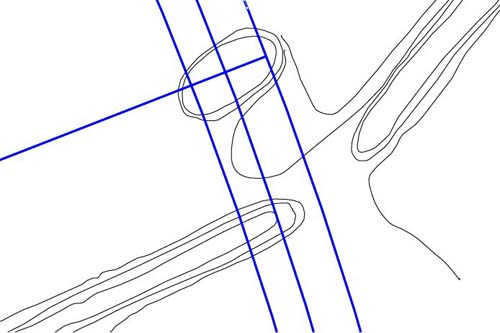Figure 87: The most central position of the mound sitting adjacent to the SE gateway complies to a distance of 2222 feet from the altar station. This is similar to the 111.111 azimuth code of Alligator Mound a few miles to the west and was probably meant to convey 2222.2222.

Other plausible readings on the small, inner Octagon mound would include 2200 feet (navigation) and 2250 feet (22.5-degrees was an important segmentation within 360-degrees). The azimuth angle from the altar station was 68.75-degrees (a mathematical progression stemming from this was usable within the "11" series navigational system.

• The value 2200 feet is 400 fathoms or 26400 inches.

CONTINUE